# 12 MM CEMENT PLASTER CALCULATOR

## 12 MM CEMENT PLASTER CALCULATOR

### Material Calculation

Volume of Mortar = Area of Plaster x Thickness of Plaster

 Note – Add 20% to fill up Joint and Cover the Surface

 Volume of Mortar of 12 mm thick Cement Plaster (For 100 m2  Surface)  = 1.44 m2

#### (A) For 12mm Cement Plaster (1:6)

Note – Increases by 25% of the Total Dry Volume
————(For 100 m2 Plaster Area)

For 1 m2 Plaster Area = 1.80/100 = 0.018 m2 Dry Volume of Mortar Needed

 Dry Volume of Mortar  of 12 mm thick Cement Plaster (For 1 m2  Surface)  = 0.018 m2

#### Step 1: Amount of Cement Required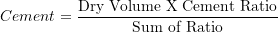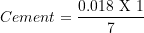Cement Required = 0.00257 m3

#### Step 2: Quantity of Coarse Sand Required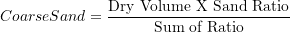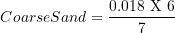Coarse Sand Required = 0.01542 m3

Note: By considering dry density of sand = 1550 kg/m3

#### Volume of Mortar = 1.44 m2

For Cement Mortar (1:5)

 Note – Increases by 28.4% of the Total Dry Volume Cement Mortar (1:5) According to BCD Analysis Rates 2114 Cement Mortar (1:5) Corse Sand = 1.07 m3 Cement Required = 0.214 m3 (1.07/5) Then Total Volume = 1.07+0.214 = 1.284 m3

————(For 100 m2 Plaster Area)

For 1 m2 Plaster Area = 1.8489/100 = 0.018489 m2 Dry Volume of Mortar Needed

#### Step 1: Amount of Cement Required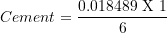Cement Required = 0.00308 m3

#### Step 2: Quantity of Coarse Sand Required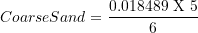Coarse Sand Required = 0.01541 m3

Note: By considering dry density of sand = 1550 kg/m3

#### Volume of Mortar = 1.44 m2

For Cement Mortar (1:4)

 Note – Increases by 34% of the Total Dry Volume Cement Mortar (1:4) According to BCD Analysis Rates 2114 Cement Mortar (1:4) Corse Sand = 1.07 m3 Cement Required = 0.2675 m3 (1.07/4) Then Total Volume = 1.07+0.2675 = 1.3375 m3

————(For 100 m2 Plaster Area)

For 1 m2 Plaster Area = 1.9296/100 = 0.019296 m2 Dry Volume of Mortar Needed

#### Step 1: Amount of Cement Required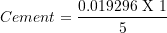Cement Required = 0.003859 m3

#### Step 2: Quantity of Coarse Sand Required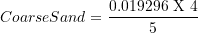Coarse Sand Required = 0.01541 m3

Note: By considering dry density of sand = 1550 kg/m3

#### Volume of Mortar = 1.44 m2

For Cement Mortar (1:3)

 Note – Increases by 42.7% of the Total Dry Volume Cement Mortar (1:4) According to BCD Analysis Rates 2114 Cement Mortar (1:3) Corse Sand = 1.07 m3 Cement Required = 0.357 m3 (1.07/3) Then Total Volume = 1.07+0.357 = 1.427 m3

————(For 100 m2 Plaster Area)

For 1 m2 Plaster Area = 2.055/100 = 0.02055 m2 Dry Volume of Mortar Needed

#### Step 1: Amount of Cement Required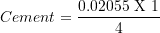Cement Required = 0.00514 m3

#### Step 2: Quantity of Coarse Sand Required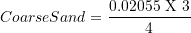Coarse Sand Required = 0.01541 m3

Note: By considering dry density of sand = 1550 kg/m3

#### For 12 mm Cement Plaster (1:3) of 1 m2 Plaster Area

Cement Required = 0.00514 m3

Coarse Sand Required = 0.01541 m3

For Floating Coat of Neat Cement  2kg/mCement Will Be Added

#### Step 1: Amount of Cement Required

Cement = 0.00514+0.0014 = 0.00654 m3

#### Step 2: Quantity of Coarse Sand Required

Same As Above

Coarse Sand Required = 0.01541 m3

#### For 12 mm Cement Plaster (1:4) of 1 m2 Plaster Area

Cement Required = 0.003859 m3

Coarse Sand Required = 0.01541 m3

For Floating Coat of Neat Cement  2kg/mCement Will Be Added

#### Step 1: Amount of Cement Required

Cement = 0.003859+0.0014 = 0.00526 m3

#### Step 2: Quantity of Coarse Sand Required

Same As Above

Coarse Sand Required = 0.01541 m3

Updated: August 12, 2023 — 1:14 pm